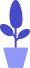# Python function calls and return values

Estimated reading: 2 minutes 55 views

Defining a function only gives the function a name, specifying the parameters and code block structure contained within the function. After the basic structure of the function is completed, it can be executed through another function call. This tutorial will talk about the call and return of the function.

## How to call functions in Python

When calling a function, you need to follow the function name with a pair of parentheses, and then put the parameters used when calling the function in parentheses.

Let’s call the function created in the previous section. In parentheses after the name of the function firstFunction, is the parameter “World” to be passed in. We represent function calls with highlighted lines of code as follows.

``````def firstFunction(name):
str1="Hello "+name+"!"
print(str1)
firstFunction("World")``````

The results obtained are shown in Figure 1.figure 1

## Return value of Python function

The return value is the value output by the function, which we can use elsewhere in the code. A function may or may not have a return value. In the previous example, when we called the function, the function did not return a value. But sometimes, we need to let the function give a return result.

Taking the sum function in the previous tutorial as an example, this time we don’t print anything in the function, but use the keyword return to return the calculation result to the function call. The code is as follows.

``````def sum(number1,number2):
result=number1+number2
return result``````

When calling this function, you can assign the return value of this function to a variable and print the variable.

``````s=sum(12,21)
print(str(s))``````

Running this code yields the result shown in Figure 2.figure 2